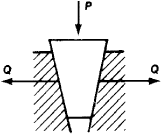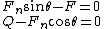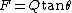# wedge

(redirected from wedge-like)
Also found in: Dictionary, Thesaurus, Medical, Financial.

## wedge,

piece of wood or metal thick at one end and sloping to a thin edge at the other; an application of the inclined planeinclined plane,
simple machine, consisting of a sloping surface, whose purpose is to reduce the force that must be applied to raise a load. To raise a body vertically a force must be applied that is equal to the weight of the body, i.e.
. It is employed in separating two objects from each other or in separating one part of a solid object from an adjoining part, as in splitting wood. The thin edge is applied to the surface of the solid or to the crack between two solids, and force is applied to the opposite thick edge. The ax, chisel, knife, nail, and carpenter's plane are wedges, and the camcam,
mechanical device for converting a rotating motion into a reciprocating, or back-and-forth, motion, or for changing a simple motion into a complex one. A simple form of cam is a circular disk set eccentrically on a shaft in order to induce (when the shaft rotates) a rising
is a rotating wedge.
The following article is from The Great Soviet Encyclopedia (1979). It might be outdated or ideologically biased.

## Wedge

a part shaped like a prism, with two working surfaces that converge at an angle (see Figure 1). The cleaving action of a wedge provides a substantial force advantage: at a small angle and a rated coefficient of friction of 0.1, the transverse force Q can reach 5P, where P is the longitudinal force applied to the wedge. The force Q is usually assumed to be 1.25P to 1.5P.Figure 1. Action of forces in a joint containing a wedge

Wedges are used to produce cleaving and cutting effects (for example, in chipping off metal parts or splitting firewood) or as an adjusting element (to provide clearance in the guides for rectilinear movement in machine tools) and also to clamp parts or, less frequently, to transmit reciprocating motion at an angle.

## wedge

[wej]
(communications)
A convergent pattern of equally spaced black and white lines, used in a television test pattern to indicate resolution.
(design engineering)
A piece of resistant material whose two major surfaces make an acute angle.
(electromagnetism)
A waveguide termination consisting of a tapered length of dissipative material introduced into the guide, such as carbon.
(engineering)
In ultrasonic testing, a device which directs waves of ultrasonic energy into the test piece at an angle.
(mathematics)
A polyhedron whose base is a rectangle and whose lateral faces consist of two equilateral triangles and two trapezoids.
(meteorology)
(optics)
An optical filter in which the transmission decreases continuously or in steps from one end to the other.
A refracting prism of very small angle, inserted in an optical train to introduce a bend in the ray path.
McGraw-Hill Dictionary of Scientific & Technical Terms, 6E, Copyright © 2003 by The McGraw-Hill Companies, Inc.

## Wedge

A piece of resistant material whose two major surfaces make an acute angle. It is closely related to the inclined plane and is used to multiply the applied force and to change the direction in which it acts (see illustration).

Force F is the smaller applied force and Q is the larger force to be exerted. In the absence of friction, forces must act normal to their surfaces; thus the actual force on the inclined surface is not Q but a larger force Fn. Summing up forces in the horizontal and vertical directions gives Eqs. (1). (1)Combining the expressions for F and Q and solving for F gives Eq. (2).

(2)If angle Θ is small, the reaction of Q against F is exceeded by the friction between the face of the wedge and the adjacent body on which it rests. Thus the wedge tends to remain in position even when loaded by a large force Q. See Simple machine

McGraw-Hill Concise Encyclopedia of Engineering. © 2002 by The McGraw-Hill Companies, Inc.

## wedge

1. A piece of wood, metal, or other hard material, thick at one end and tapering to a thin edge at the other.